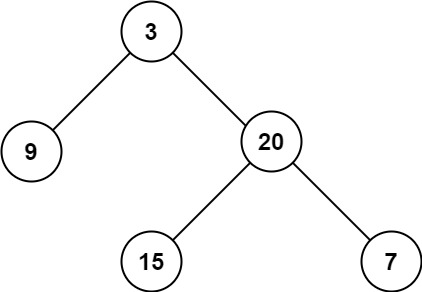엔지니어 게시판
LeetCode 솔루션 분류

# [Easy - wk4 - Q3] 104. Maximum Depth of Binary Tree

• Chloe 작성
• 작성일

• 419 조회
• 3 댓글

### 본문

Given the `root` of a binary tree, return its maximum depth.

A binary tree's maximum depth is the number of nodes along the longest path from the root node down to the farthest leaf node.

Example 1:```Input: root = [3,9,20,null,null,15,7]
Output: 3
```

Example 2:

```Input: root = [1,null,2]
Output: 2
```

Constraints:

• The number of nodes in the tree is in the range `[0, 104]`.
• `-100 <= Node.val <= 100`

댓글 3

## mingki님의 댓글

• mingki
• 작성일
C++
Runtime: 12 ms, faster than 55.34% of C++ online submissions for Maximum Depth of Binary Tree.
Memory Usage: 18.9 MB, less than 14.43% of C++ online submissions for Maximum Depth of Binary Tree.
``````class Solution {
public:
int maxDepth(TreeNode* root) {
if (!root) return 0;
return max(maxDepth(root->left), maxDepth(root->right)) + 1;
}
};``````

## dawn27님의 댓글

• dawn27
• 작성일
JS
Runtime: 76 ms, faster than 78.73% of JavaScript online submissions for Maximum Depth of Binary Tree.
Memory Usage: 45 MB, less than 63.27% of JavaScript online submissions for Maximum Depth of Binary Tree.
``````var maxDepth = function(root) {
let counter = 0
let BFS = (root, level) => {
if (!root) return;
if (counter < level) {
counter = level;
}
BFS(root.left, level+1);
BFS(root.right,level+1);
}
BFS(root, 1);
return counter;
}``````

## Jack님의 댓글

• Jack
• 작성일
Java

``````class Solution {
public int maxDepth(TreeNode root) {
if (root == null) {
return 0;
} else {
int left_height = maxDepth(root.left);
int right_height = maxDepth(root.right);
return java.lang.Math.max(left_height, right_height) + 1;
}
}
}``````
전체 126 / 1 페이지

### 새댓글

• 등록자 JakeMinSVK 등록일 18:25
• 등록자 JakeMinSVK 등록일 18:24
• 등록자 학부유학생 등록일 06.22
• 등록자 학부유학생 등록일 06.21
• 등록자 Coffee 등록일 06.21
• 등록자 Coffee 등록일 06.21
• 등록자 SVKOREANS 등록일 06.20
• 등록자 학부유학생 등록일 06.20
• 등록자 Coffee 등록일 06.20
• 등록자 키위 등록일 06.20